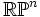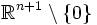# Real projective space

Jump to: navigation, search

## Definition

Real projective space of dimension$n$, denoted as$\R\mathbb{P}^n$, is defined as the quotient of the set$\R^{n+1}\setminus \{ 0 \}$ via the group action of$\R^*$ by scalar multiplication. Equivalently, it is the set of all directions in$\R^{n+1}$, suitably topologized.

It can also be viewed as the quotient of the$n$-dimensional sphere via identification of antipodes. This is because$S^n$ (the$n$-sphere is the quotient of$\R^{n+1}\setminus \{ 0 \}$ under the action of the multiplicative group of positive real numbers, which is a normal subgroup of index two in the multiplicative group of all nonzero real numbers.

## Geometric properties

### Metric structure

The real projective space can be viewed as a Riemannian manifold when equipped with the Fubini-Study metric.

### Curvature

The Fubini-Study metric is a constant-curvature metric. This constant curvature is negative for even dimensions and positive for odd dimensions.

## Automorphisms and symmetries

### Homogeneousness

Real projective spaces are homogeneous, in the sense that given any two points in the real projective space, there is a Riemannian isometry taking one to the other.